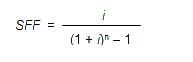# Sinking Fund Method

Sinking fund method is a method of calculating depreciation for an asset in which apart from calculating depreciation, it also keeps aside a fund for replacing the asset at the end of its useful life.

This method is used when the assets that need to be replaced are of high cost. To avoid paying for the replacement of assets at a time, companies maintain a sinking fund that will help them recover the cost of the asset while also accounting for its depreciation.

Sinking fund method is put into use by large scale industries such as utility industries that have a requirement for expensive long-term assets to function.

## How Sinking Fund Functions

Sinking fund functions as an account where the company stores funds in anticipation that it will become dysfunctional after a certain point of time. Instead of expenditure that will arise at a time which can cause a significant impact on company’s profit, it is better that such an expense can be anticipated and the funds for replacement of such an asset can be made wisely.

Under the sinking fund method, the depreciation that is charged for the asset is transferred to a sinking fund account. The same amount is then invested in securities issued by the government, interest that is earned on such securities are reinvested.

The interest that is earned on the amount invested as depreciation will also be invested and will be invested to the extent of the useful life of the asset. When the asset needs replacement, the investment which is done in the form of securities are sold, and the asset will be purchased from the amount thus obtained.

Also, the old assets book value will be deposited to the Sinking fund account. The value obtained by selling off an old asset, which can be either profit or loss is then moved to the Sinking Fund Account.

The balance of the sinking fund account is then further transferred to General Reserve. The sinking fund account is also known as depreciation fund account.

## Sinking Fund Formula

The formula for sinking fund is as follows.Where

A = Money Accumulated

P = Periodic Contribution to the sinking fund

r = Rate of Interest

n = number of years

m = number of payments per year

The periodic contribution can be calculated with the following formula## Sinking Fund Benefits

Following are the benefits of maintaining a sinking fund:

1. It will improve the company finances: As the economic situation of a company will not be the same, the need for replacing a high value asset can be detrimental to the company’s financial position. By having a sinking fund, the company can cover those expenses, and it will keep the investors confident about the company.

2. Brings Investment: Companies with a high level of debt are deemed as risky, but a sinking fund instils confidence in the investors and can bring in further investment.

3. Lower Interest Rates: Companies with a sinking fund can get investment for low interest rates.

This was all about Sinking Fund Method. The concept presented in this article will be helpful for students in developing the knowledge of the Sinking Fund, and it’s uses. For more such interesting concepts, stay tuned to BYJU’S.

## FAQ’S

1. How sinking fund is calculated

Sinking fund is calculated by the formulaWhere

A = Money Accumulated

P = Periodic Contribution to the sinking fund

r = Rate of Interest

n = number of years

m = number of payments per year

2. What is the most notable feature of the sinking fund method of depreciation?

The most notable feature of Sinking fund method of depreciation is that it keeps funds for replacement of an asset after its useful life.

3. How to calculate sinking fund factor

Sinking fund factor can be calculated by the following formula